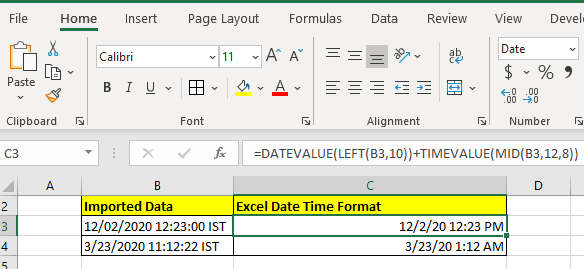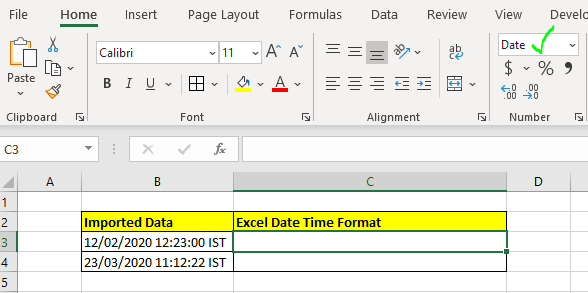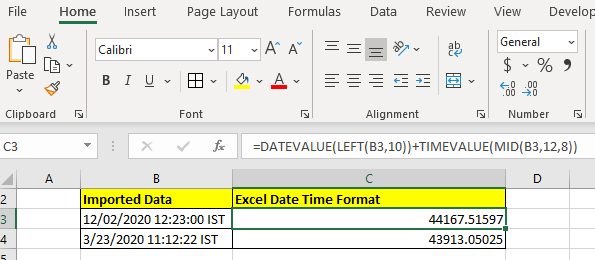# How to Convert String To Excel DatetimeIt is common to import data from other sources in Excel and it is also common to not get properly formatted data. For example, most of the time when we get data from other sources the date, time and numeric values are in text format. They need to be converted in the proper format. It is easy to convert numbers into excel numbers. But converting string into excel datetime may need a little bit of effort.

In this article, we will learn how you can convert text formatted date and time in excel date and time.

Generic Formula

 =DATEVALUE(LEFT(Datetime_string,10)) + TIMEVALUE(MID(Datetime_string,12,8))

Datetime_string: This is the reference of the string that contains the date and time.

The main functions in this generic formula are DATEVALUE and TIMEVALUE. Other functions are used to extract date string and time string if they are combined into one string. And yes, the cell that contains this formula should be formatted into datetime format otherwise you will see serial numbers that may confuse you.

Let's see some examples to make things clear.

## Example: Convert Imported Date and Time into Excel DatetimeHere we have date and time combined into one string. We need to extract date and time separately and convert them into excel datetime.

Note that I have formatted C3 as a date so that the results I get are converted into dates and I don't see a fractional number. (Secrets of excel date and time).

So let's write the formula:

 =DATEVALUE(LEFT(B3,10)) + TIMEVALUE(MID(B3,12,8))

And it returns the date and time that excel considers as datetime, not string.If you don't change the cell formatting, this is what your result will look like.This isn't wrong. It is the serial number that represents that date and time. If you don't know how the date and time are perceived by Excel, you can get complete clarity here.

How does it work?

This is simple. We use extraction techniques to extract date and time strings. Here we have LEFT(B3,10). This returns 10 characters from the left of the string in B3. Which is "12/02/2020". Now this string is supplied to the DATEVALUE function. And DATEVALUE converts the date string into its value. Hence we get 44167.

Similarly, we get time value using  MID function (for extraction) and TIMEVALUE function.

The MID function returns 8 characters from the 12th  position in string. Which is "12:23:00". This string is supplied to TIMEVALUE function that returns .51597.

Finally we add these numbers up. This gives us the number 44167.51597. When we convert the cell formatting to MM/DD/YYYY HH:MM AM/PM format we get the exact datetime.

In the above example, we had date and time concatenated together as a string. If you just have date as string without any extra text or string, you can use the DATEVALUE function alone to get the Date converted into excel date.

 =DATEVALUE(B3)

If you just have time as a string use the TIMEVALUE function

 =TIMEVALUE(B3)

You can read about these functions by clicking on the function name in the formula.

So yeah guys, this is how you convert a date and time string into excel date time value. I hope this was helpful. If you have any doubts regarding this article or have any other query related Excel/VBA, ask me in the comment section below. I will be happy to help you.

Related Articles:

Convert Date and Time from GMT to CST : While working on Excel reports and Excel dashboards in Microsoft Excel, we need to convert Date and Time. In addition, we need to get the difference in timings in Microsoft Excel.

How to Convert Time to Decimal in Excel : We need to convert the time figures into decimals using a spreadsheet in Excel 2016. We can manipulate time in excel 2010, 2013 and 2016 using the function CONVERT, HOUR and MINUTE.

How to Convert date to text in Excel : In this article we learned how to convert text into date, but how do you convert an excel date into text. To convert an excel date into text we have a few techniques.

Converting Month Name to a Number in Microsoft Excel : When it comes to reports numbers are better than text. In Excel if you want to convert month names into numbers (1-12). Using the function DATEVALUE and MONTH you can easily convert months into numbers.

Excel convert decimal Seconds into time format : As we know, time in excel is treated as numbers. Hours, Minutes, and Seconds are treated as decimal numbers. So when we have seconds as numbers, how do we convert them into time format? This article got it covered.

Popular Articles:

50 Excel Shortcuts to Increase Your Productivity | Get faster at your task. These 50 shortcuts will make you work even faster on Excel.

How to use Excel VLOOKUP Function| This is one of the most used and popular functions of excel that is used to look up value from different ranges and sheets.

How to use the Excel COUNTIF Function| Count values with conditions using this amazing function. You don't need to filter your data to count specific values. Countif function is essential to prepare your dashboard.

How to Use the SUMIF Function in Excel | This is another dashboard essential function. This helps you sum up values on specific conditions.

Terms and Conditions of use

The applications/code on this site are distributed as is and without warranties or liability. In no event shall the owner of the copyrights, or the authors of the applications/code be liable for any loss of profit, any problems or any damage resulting from the use or evaluation of the applications/code.